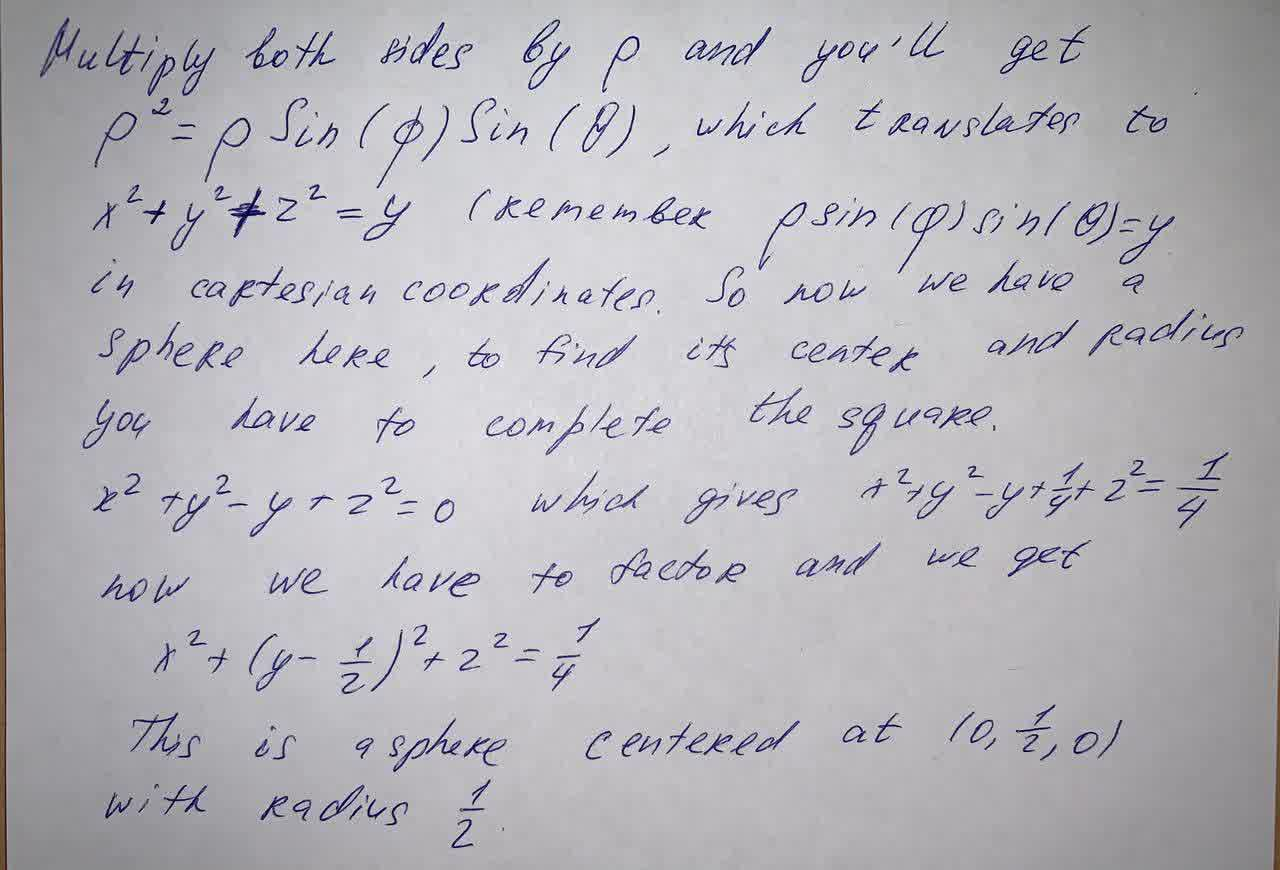Question# Identify the surface whose equation is given. \rho= \sin \theta \sin \phi

First order differential equations
ANSWEREDIdentify the surface whose equation is given.
$$\rho= \sin \theta \sin \phi$$2021-06-012021-09-09

Multiply both sides by p and you'll get $$p^2=p\sin(\phi)\sin(\theta)$$, which translates to $$x^2+y^2+z^2=y$$, remember $$p\sin(\phi)\sin(\theta)=y$$ in cartesian coordinates,

So now we have a sphere here, to find its center and radius you have to complete the square.

$$x^2+y^2-y+z^2=0$$ which gives $$x^2+y^2-y+\frac{1}{4}+2^2=\frac{1}{4}$$ now we have to factor and we get $$x^2+(y-\frac{1}{2})^2+2^2=\frac{1}{4}$$

This is a sphere centered at $$(0,\frac{1}{2},0)$$ with radius $$\frac{1}{2}$$